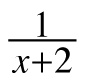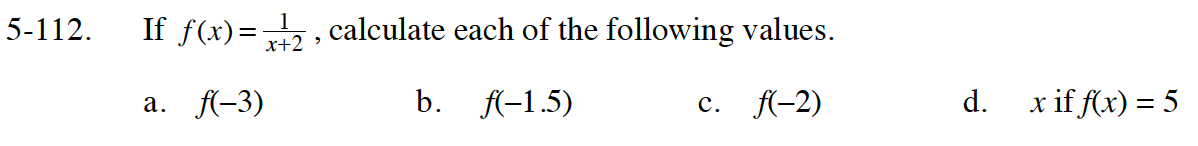Home > INT1 > Chapter Ch5 > Lesson 5.3.2 > Problem5-112

5-112.
1. If f(x) =, calculate each of the following values. Homework Help ✎

1. f(–3)

2. f(–1.5)

3. f(–2)

4. x if f(x) = 5Evaluate the fraction when x = -3

$f(-1.5)=\frac{1}{-1.5+2}$

$f(-1.5)=\frac{1}{0.5}$

Undefined.
Since evaluating the function at −2 results in a zero in the denominator, the function is undefined at x = −2

$\text{Solve the equation: }$

$5=\frac{1}{\textit{x}+2}$